# Introduction to AMOS

## IBM SPSS AMOS Series - Introduction to AMOS.

The session discusses

• What is AMOS?
• Understanding Diagram Symbols
• AMOS Terminologies
• Sample Model in AMOS and What it Means?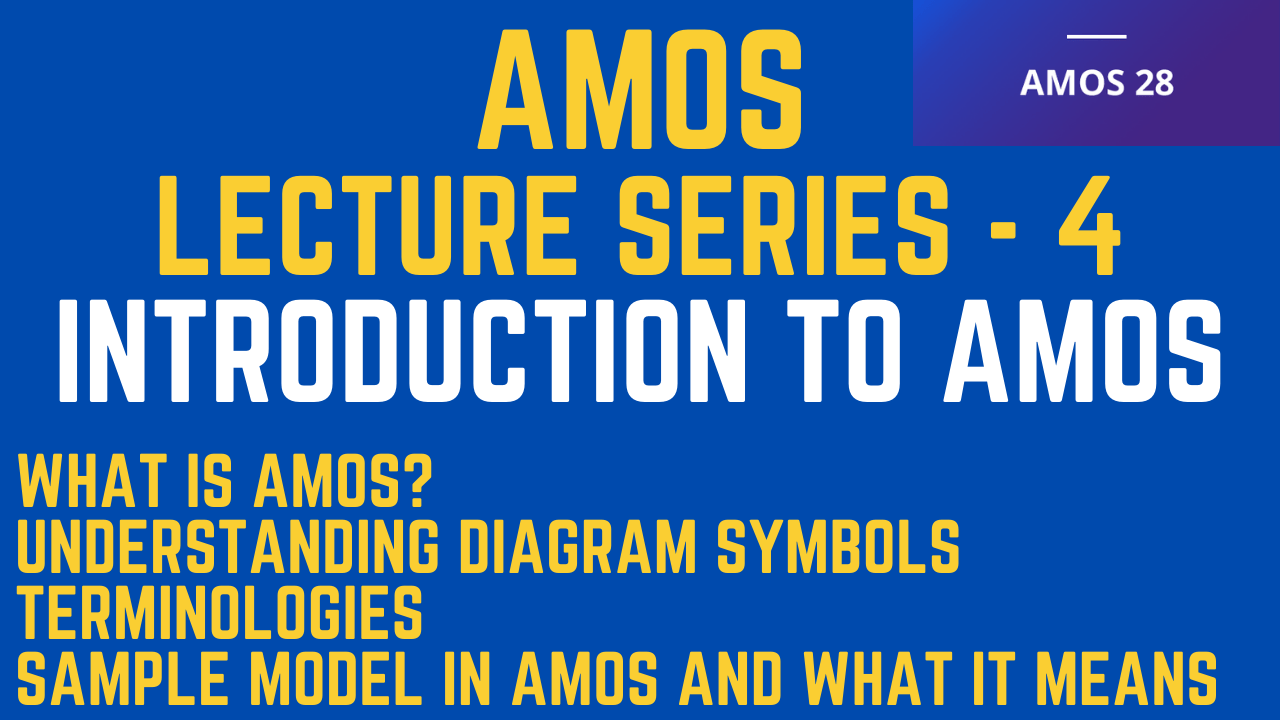### Introduction to AMOS

The session discusses the very basics of AMOS including the different concepts, terminologies, and a sample AMOS model

## What is AMOS?

• AMOS stands for “Analysis of Moment Structures”. Moment structures refer to means, variance, and covariance.
• AMOS is a program tied to SPSS that uses a graphical interface for input.
• AMOS software could be utilized to explore statistical relationships among the items of each construct and also between constructs.
• Using AMOS, the researcher can specify, estimate, assess, and present the model in a causal path diagram to show the hypothesized relationships among constructs of interest.
• The empirical model can be tested against the hypothesized model for goodness of fit.
• If the researchers found any path that does not fit with the original model, they could either modify the path to improve the fitness of the model or remove that particular path completely from the hypothesized model.

## Understanding the Diagram Symbols

• SEM uses diagrams to denote relationships to be tested.
• It is important that you understand what these diagram symbols mean because AMOS is going to make you draw out your conceptual model.
• One of the frustrating aspects of SEM is that there are often multiple terms that mean the exact same thing.
• You can read three different books, and all three can use different terms to mean the same thing. Hence, all the overlapping terms here to add greater clarity to the different nomenclature.

## Terminologies and Symbols

• Latent Variable/Construct—A latent variable is also referred to as “unobservable”.
• This is a concept that cannot be directly observed. It would be nice to just look at someone and tell his/her level of anxiety, but the fact is some people are great at hiding their true feelings.
• In these instances, we cannot simply observe a person and determine levels of anxiety. Thus, concepts such as anxiety are an “unobservable” and require the researcher to find a way to capture the concept by other means, such as asking survey questions.
• You will also see the term “Factors” used when referring to latent/unobservable constructs. Examples of unobservable constructs in psychology are Anxiety, Motivation, and Trust.
• These unobservable constructs are often measured by “indicators”, or “measurement items”, which often take the form of survey questions. For example, you might ask survey questions (indicators) to measure a consumer’s level of trust with a company (unobservable).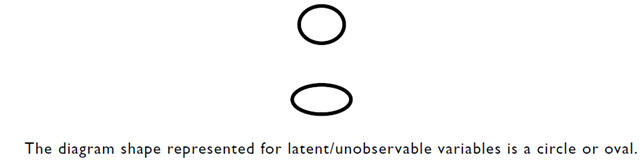• Observed Variable/Indicator—As the name denotes, measures are taken to capture an unobservable concept through observable means.
• This can be done through survey questions, manipulations, or behavioral tracking.
• This concept is also referred to as Manifest Variables or Reference Variables. You will often see the term “items” and “indicators” used to represent observed variables.
• In essence, observed variables/indicators are the raw data captured that will be used to explain concepts in a SEM model. These observed variables/indicators can be categorical, ordinal, or continuous.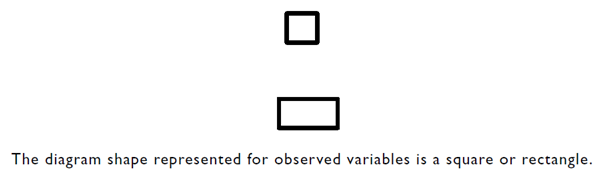• Measurement Error/Residual Term—Measurement error represents the unexplained variance by an indicator measuring its respective latent construct.
• In trying to capture an unobservable construct with a measurement indicator, the unexplained variance in the measurement is error, or “measurement error”. Along with the indicator, an error term is also present on a dependent latent construct.
• This is the unexplained variance on the construct level as a result of the relationships from the independent variables.
• Error terms for latent variables are also called residual terms or disturbance terms. Since measurement error and residual terms represent unexplained variance, AMOS treats these terms like unobserved variables.
• Thus, the symbol for these error terms are a circle and a one-way arrow.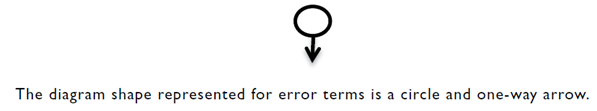Direct Path Effect—Hypothesized directional effects of one variable on another.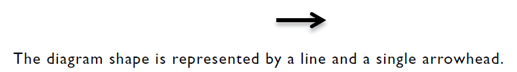Covariances—the amount of change in one variable that is consistently related to the change in another variable (the degree to which the two variables change together on a reliable and consistent basis).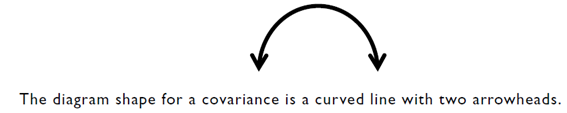Independent vs. Dependent Latent Variables

Independent variables (also called exogenous variables) are the constructs that influence another variable. Dependent variables (called endogenous variables) are constructs influenced by independent variables.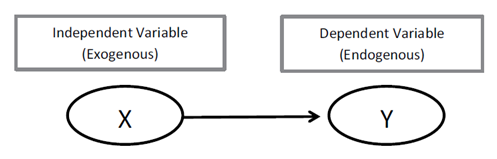## Sample AMOS Model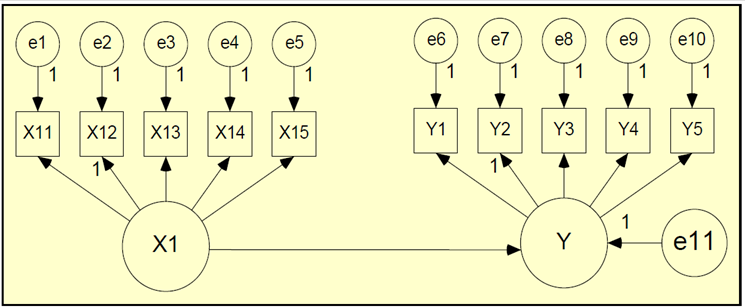• Note: X1 and Y are latent constructs. In Amos syntax, latent constructs are represented by the ellipses. The latent construct X1 is measured using items X11 to X15, while latent construct Y is measured using items Y1 to Y5. The measured items are represented by rectangles in the model. Sometimes (in literature) the measured items are called latent indicators.
• Key: X1 = Exogenous construct, while X11 to X15 is a set of 5 items to measure latent construct X1
• In the Amos diagram, e1 to e5 are errors in measurement for items X11 to X15
• Y = Endogenous construct, while Y1 to Y5 is a set of 5 items to measure latent construct Y
• In the Amos diagram, e6 to e10 are errors in measurement for items Y1 to Y5

## References

• Collier, J. E. (2020). Applied structural equation modeling using AMOS: Basic to advanced techniques. Routledge.
• Awang, Z. (2015). A Handbook on SEM (2nd Edition). Malaysia